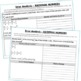# RATIONAL NUMBERS (Fractions and Decimals) Error Analysis (Find the Error)Subject
Resource Type
File Type

PDF

(924 KB|14 pages)
Standards
Also included in:
1. I use this RATIONAL NUMBERS Unit Resource BUNDLE every year with my students. This BUNDLE includes 40 task cards, 10 error analysis activities,10 problem solving graphic organizers, 6 practice worksheets, 5 guided notes, 1 maze, 1 riddle, and 1 color by number page (over 165 skills practice and real
\$17.50
\$14.00
Save \$3.50
2. Have your students engage in higher order thinking with these math ERROR ANALYSIS activities. This GROWING BUNDLE resource includes 8 different sets (77 questions) of 7th Grade Common Core word problems that are solved incorrectly. Students have to IDENTIFY THE ERROR, provide the CORRECT SOLUTION a
\$24.00
\$19.20
Save \$4.80
3. - Great for online learning and distance learning!This middle school ERROR ANALYSIS BUNDLE includes 230 real-world word problems (23 sets) that are solved incorrectly for grades 6-8. Students have to IDENTIFY THE ERROR, provide the CORRECT SOLUTION and share a helpful STRATEGY for solving the proble
\$69.00
\$48.30
Save \$20.70
• Product Description
• Standards
Have your students apply their understanding of RATIONAL NUMBERS with this ERROR ANALYSIS activities.

Benefits of Math Error Analysis:
Giving students opportunities to identify and correct errors in presented solutions allows them to show their understanding of the mathematical concepts you have taught.

Whats Included:
This resource includes 10 real-world RATIONAL NUMBERS word problems that are solved incorrectly. Students have to IDENTIFY THE ERROR, provide the CORRECT SOLUTION and share a helpful STRATEGY for solving the problem. An ANSWER KEY has also been provided with examples of possible answers. Be sure to download the sample for a full overview of what you get.

How to Use:
When I present this activity to my students, I tell them that these are errors that students from my other class made and I need their help to correct them. Students LOVE correcting other students mistakes. Students can use these activities with a partner, as a warm-up , as classwork, homework, in math centers or group work.

Topics included:
✔ adding, subtracting, multiplying, dividing positive and negative FRACTIONS
✔ adding, subtracting, multiplying, dividing positive and negatives DECIMALS

This resource is included in my RATIONAL NUMBERS UNIT RESOURCE BUNDLE!

Common Core Alignment :
*7.NS.1 -Apply and extend previous understandings of addition and subtraction to add and subtract rational numbers;
*7.NS.2 - Apply and extend previous understandings of multiplication and division and of fractions to multiply and divide rational numbers.
*7.NS.3 - Solve real-world and mathematical problems involving the four operations with rational numbers.
*Mathematics Practice Standard 3 (construct viable arguments and critique the reasoning of others).

FEEDBACK on this resource:
♥ My students loved this activity. Created such good discussions between my students.
♥ Love these, great for stations!
♥ One of the things my students struggle with most is identifying the error(s) in their own or someone else's work. This provides great practice on that skill.
♥ Great for higher level thinking! Thanks!

More RATIONAL NUMBER resources:
* ERROR ANALYSIS
* PROBLEM SOLVING INTERVENTION
* PRACTICE WORKSHEETS
* MAZE, RIDDLE & COLORING PAGE ACTIVITIES

BUNDLE UP & Save \$\$ This resource is included in my:
__ Rational Numbers Reasoning RESOURCE BUNDLE
__ 7th Grade Math MEGA BUNDLE
__ 7th Grade Math Error Analysis BUNDLE
__ Middle School Math GROWING MEGA BUNDLE

If you would like to get updates on NEW and CURRENT resources...
FOLLOW the Exceeding the Core BLOG!
FOLLOW me on Teachers Pay Teachers!

© Exceeding the CORE. All rights reserved. Please note - this resource is for use by one teacher only. Additional teachers must purchase their own license. Copying, editing, selling, redistributing, or posting any part of this product on the internet is strictly forbidden. Violations are subject to the penalties of the Digital Millennium Copyright Act.

Click HERE to become a Seller on TPT. { Referral by Exceeding the CORE}
Solve real-world and mathematical problems involving the four operations with rational numbers.
Convert a rational number to a decimal using long division; know that the decimal form of a rational number terminates in 0s or eventually repeats.
Apply properties of operations as strategies to multiply and divide rational numbers.
Understand that integers can be divided, provided that the divisor is not zero, and every quotient of integers (with non-zero divisor) is a rational number. If 𝘱 and 𝘲 are integers, then –(𝘱/𝘲) = (–𝘱)/𝘲 = 𝘱/(–𝘲). Interpret quotients of rational numbers by describing real-world contexts.
Understand that multiplication is extended from fractions to rational numbers by requiring that operations continue to satisfy the properties of operations, particularly the distributive property, leading to products such as (–1)(–1) = 1 and the rules for multiplying signed numbers. Interpret products of rational numbers by describing real-world contexts.
Total Pages
14 pages
Included
Teaching Duration
45 minutes
Report this Resource to TpT
Reported resources will be reviewed by our team. Report this resource to let us know if this resource violates TpT’s content guidelines.